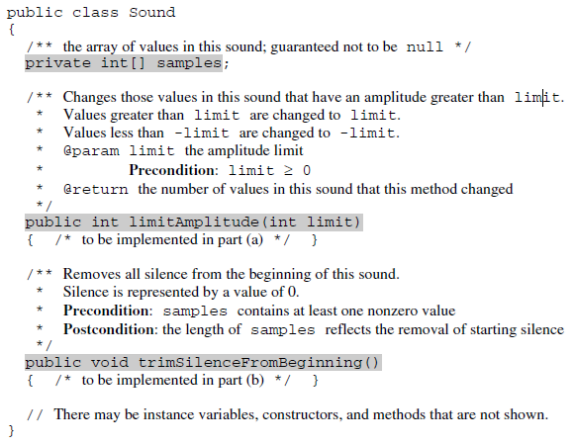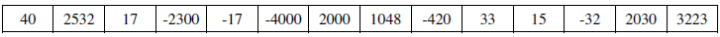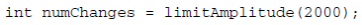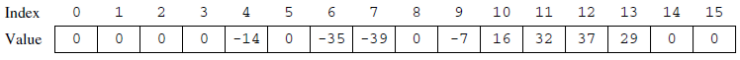# 2011 AP Computer Science A 计算机科学A真题系列之简答题免费下载

### 真题与答案下载### 2011 AP 计算机科学A简答题部分免费下载

#### 部分真题预览：

1）Digital sounds can be represented as an array of integer values. For this question, you will write two unrelated methods of the Sound class.
A partial declaration of the Sound class is shown below.1. The volume of a sound depends on the amplitude of each value in the sound. The amplitude of a value is its absolute value. For example, the amplitude of -2300 is 2300 and the amplitude of 4000 is 4000.
Write the method limitAmplitude that will change any value that has an amplitude greater than the given limit. Values that are greater than limit are replaced with limit, and values that are less than -limit are replaced with –limit. The method returns the total number of values that were changed in the array. For example, assume that the array samples has been initialized with the following values.When the statementis executed, the value of numChanges will be 5, and the array samples will contain the following values.2. Recorded sound often begins with silence. Silence in a sound is represented by a value of 0.
Write the method trimSilenceFromBeginning that removes the silence from the beginning of a sound. To remove starting silence, a new array of values is created that contains the same values as the original samples array in the same order but without the leading zeros. The instance variable samples is updated to refer to the new array. For example, suppose the instance variable samples refers to the following array.After trimSilenceFromBeginning has been called, the instance variable samples will refer to the following array.